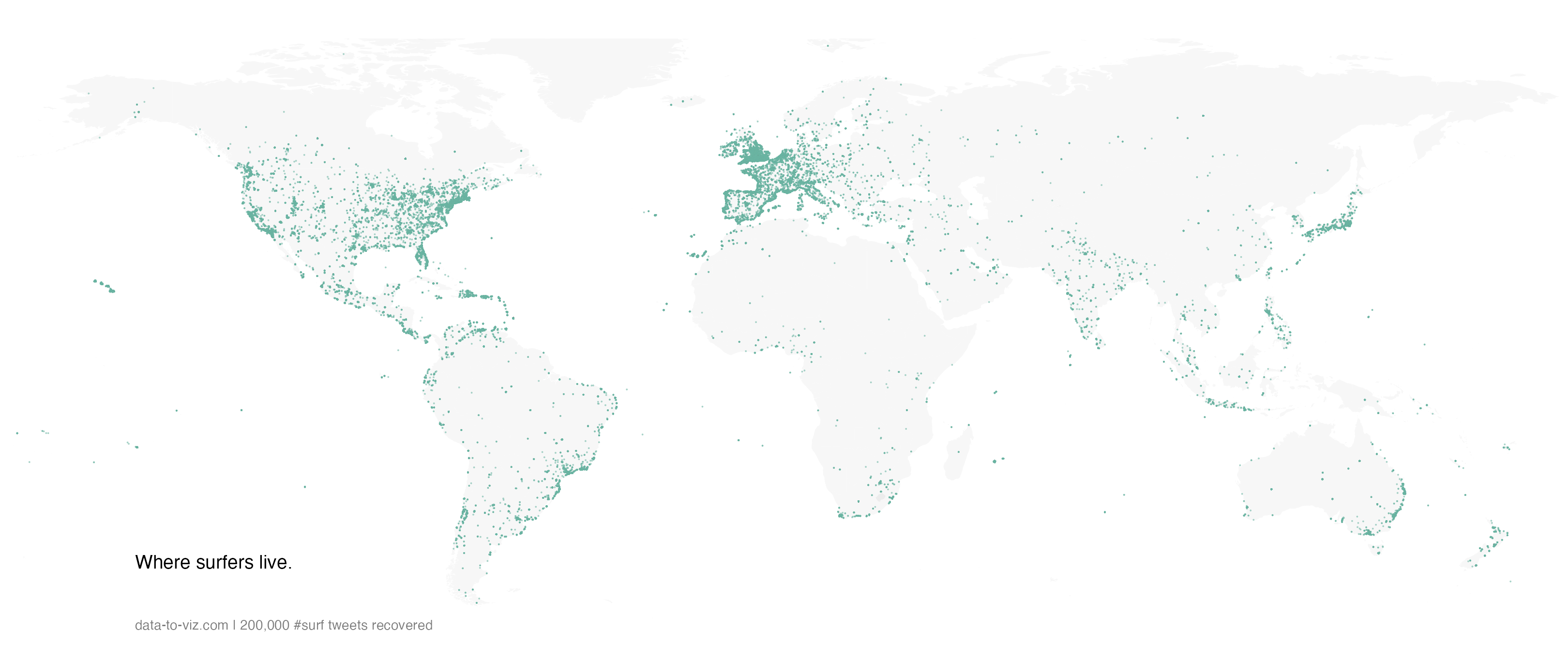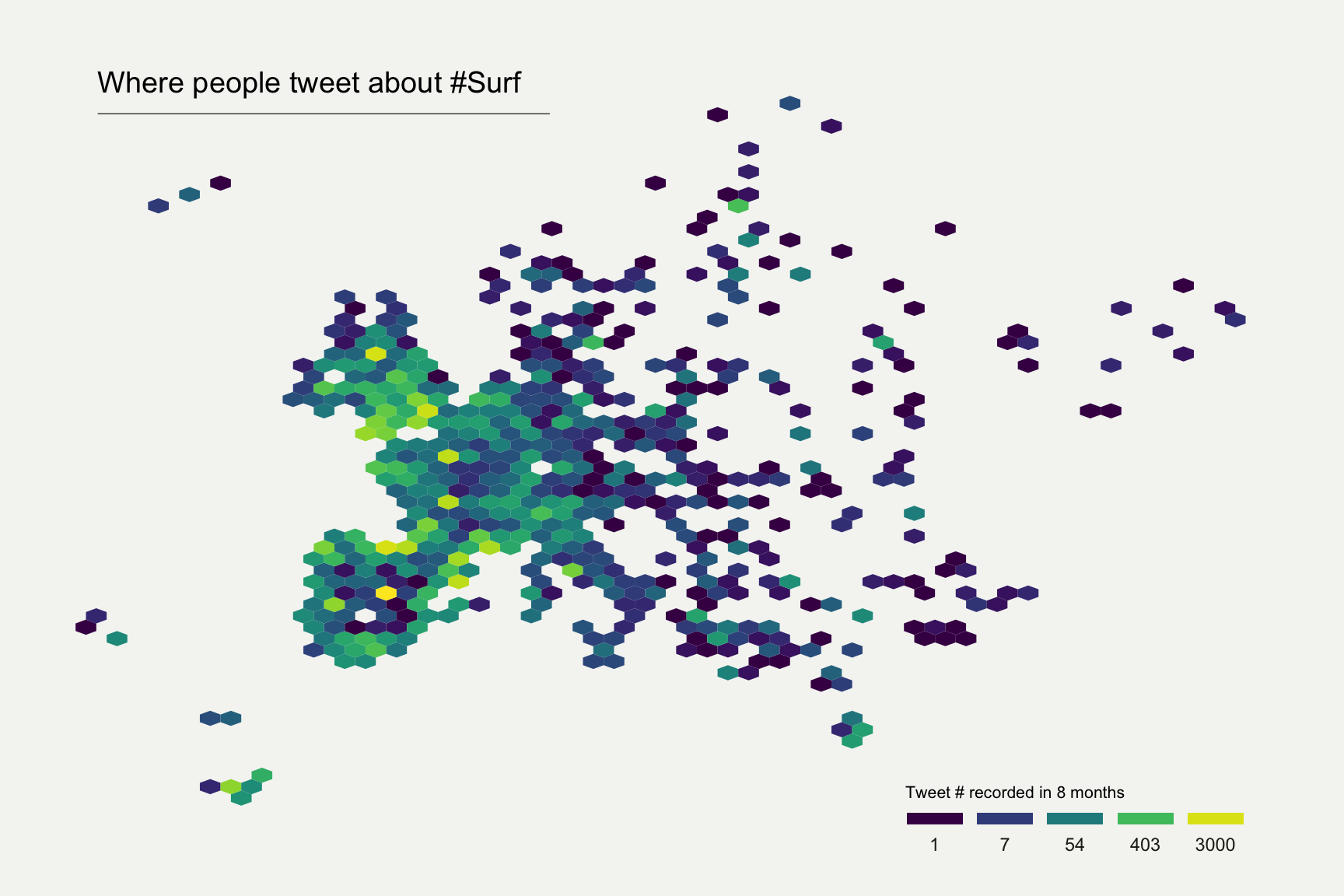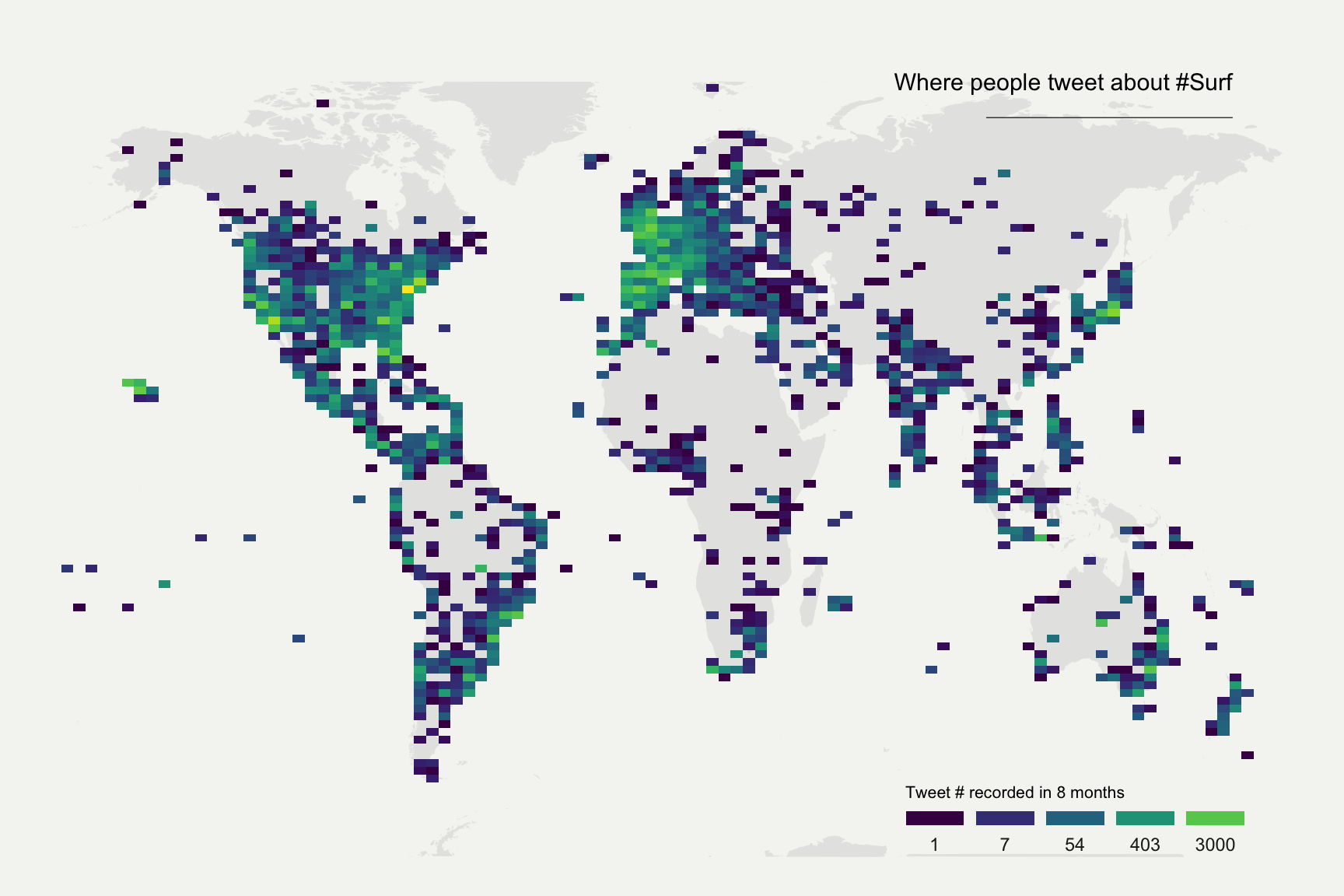This document gives a few suggestions to analyse a dataset composed by a list of GPS coordinates
It considers the geographic position of about 200k tweets containing the hashtags `#surf`, `#windsurf` or `#kitesurf`.
This dataset has been built harvesting twitter every day during about 300 days. It is fully available in this Github repository. Basically it looks like the table beside.

``````# Libraries
library(tidyverse)
library(viridis)
library(hrbrthemes)
library(kableExtra)
options(knitr.table.format = "html")
library(mapdata)

# show data
data %>% select(homelat, homelon) %>% head(3) %>% kable() %>%
kable_styling(bootstrap_options = "striped", full_width = F)``````
homelat homelon
18.28548 -70.33012
39.10312 -84.51202
19.41095 -99.27186

# Showing a few dots

If your dataset is composed by a few data point only, you can just display them on a map. If you have specific information to display concerning these positions, use an interactive: more information are available when you click data points.

# A dot density map

A dot density map is used when the sample size of your dataset is high.

``````# Get the world polygon
world <- map_data("world")

p <- data %>%
ggplot( aes(x=homelon, y=homelat)) +
geom_polygon(data = world, aes(x=long, y = lat, group = group), fill="grey", alpha=0.1) +
geom_point(size=0.8, color="#69b3a2", alpha=0.5) +
coord_equal() +
theme_void() +
theme(
panel.spacing=unit(c(0,0,0,0), "null"),
plot.margin=grid::unit(c(0,0,0,0), "cm"),
) +
ggplot2::annotate("text", x = -150, y = -45, hjust = 0, size = 11, label = paste("Where surfers live."), color = "Black") +
ggplot2::annotate("text", x = -150, y = -51, hjust = 0, size = 8, label = paste("data-to-viz.com | 200,000 #surf tweets recovered"), color = "black", alpha = 0.5) +
xlim(-180,180) +
ylim(-60,80) +
scale_x_continuous(expand = c(0.006, 0.006)) +
coord_equal()

ggsave(p, file="IMG/Surfer_position.png", width = 36, height = 15.22, units = "in", dpi = 90)``````Dotmaps give a good idea about where samples are distributed. However, once dots start to overlap, it gets impossible to distinguish how many of them are displayed on a certain zone. That’s where binning becomes an interesting option.

# Hexbin

To create a hexbin map, the territory is divided in many `hexagons` and the number of sample per hexagon is counted and represented by a color.

``````data %>%
filter(homecontinent=='Europe') %>%
ggplot( aes(x=homelon, y=homelat)) +
geom_hex(bins=59) +
ggplot2::annotate("text", x = -27, y = 72, label="Where people tweet about #Surf", colour = "black", size=5, alpha=1, hjust=0) +
ggplot2::annotate("segment", x = -27, xend = 10, y = 70, yend = 70, colour = "black", size=0.2, alpha=1) +
theme_void() +
xlim(-30, 70) +
ylim(24, 72) +
scale_fill_viridis(
trans = "log",
breaks = c(1,7,54,403,3000),
name="Tweet # recorded in 8 months",
guide = guide_legend( keyheight = unit(2.5, units = "mm"), keywidth=unit(10, units = "mm"), label.position = "bottom", title.position = 'top', nrow=1)
)  +
ggtitle( "" ) +
theme(
legend.position = c(0.8, 0.09),
legend.title=element_text(color="black", size=8),
text = element_text(color = "#22211d"),
plot.background = element_rect(fill = "#f5f5f2", color = NA),
panel.background = element_rect(fill = "#f5f5f2", color = NA),
legend.background = element_rect(fill = "#f5f5f2", color = NA),
plot.title = element_text(size= 13, hjust=0.1, color = "#4e4d47", margin = margin(b = -0.1, t = 0.4, l = 2, unit = "cm")),
) ``````Note that this is very close from an 2d histogram map. Basically, it splits the space into a set of squares instead of hexagons, and uses the same process:

``````# Make the hexbin map with the geom_hex function
ggplot(data, aes(x=homelon, y=homelat)) +
geom_polygon(data = world, aes(x=long, y = lat, group = group), fill="grey", alpha=0.3) +
geom_bin2d(bins=100) +
ggplot2::annotate("text", x = 175, y = 80, label="Where people tweet about #Surf", colour = "black", size=4, alpha=1, hjust=1) +
ggplot2::annotate("segment", x = 100, xend = 175, y = 73, yend = 73, colour = "black", size=0.2, alpha=1) +
theme_void() +
ylim(-70, 80) +
scale_fill_viridis(
trans = "log",
breaks = c(1,7,54,403,3000),
name="Tweet # recorded in 8 months",
guide = guide_legend( keyheight = unit(2.5, units = "mm"), keywidth=unit(10, units = "mm"), label.position = "bottom", title.position = 'top', nrow=1)
)  +
ggtitle( "" ) +
theme(
legend.position = c(0.8, 0.09),
legend.title=element_text(color="black", size=8),
text = element_text(color = "#22211d"),
plot.background = element_rect(fill = "#f5f5f2", color = NA),
panel.background = element_rect(fill = "#f5f5f2", color = NA),
legend.background = element_rect(fill = "#f5f5f2", color = NA),
plot.title = element_text(size= 13, hjust=0.1, color = "#4e4d47", margin = margin(b = -0.1, t = 0.4, l = 2, unit = "cm")),
) ``````# Choropleth

It is also possible to divide your territory (the world here) in regions other than square or hexagones. This will result in a chloropleth map. Of course, you need the information of the exact shape of your regions.

# Going further

You can learn more about each type of graphic presented in this story in the dedicated sections. Click the icon below: